## Mathematical Ratios Can Be Solved By Nickzom Calculator – The Calculator Encyclopedia

According to Math Is Fun,

A ratio says how much of one thing there is compared to another thing.

According to Wikipedia,

A ratio may be considered as an ordered pair of numbers, as a fraction with the first number in the numerator and the second as denominator, or as the value denoted by this fraction. Ratios of counts, given by (non-zero) natural numbers, denote positive rational numbers, and as such may occasionally result in natural numbers.

According to WikiHow,

Ratios are mathematical expressions that compare two or more numbers. They can compare absolute quantities and amounts or can be used to compare portions of a larger whole. Ratios can be calculated and written in several different ways, but the principles guiding the use of ratios are universal to all.

Nickzom Calculator+ (Professional Version) – The Calculator Encyclopedia solves ratio in mathematics for any number of fractional parts.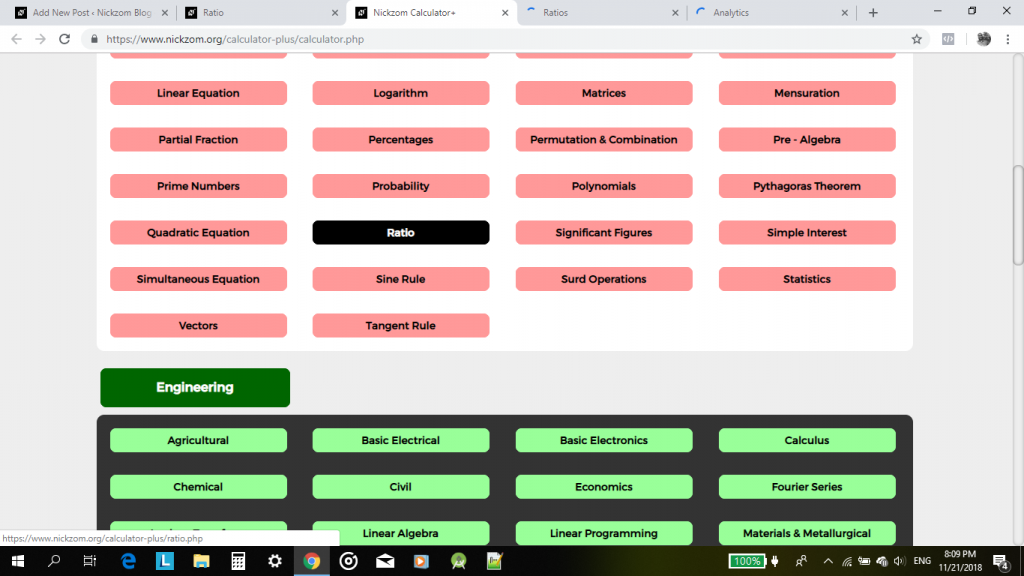For the calculator encyclopedia to be able to solve and present you a ratio. you must have to know the number of fractional parts you desire to compute the ratio for.

## Mathematical Surd Operations can be Solved by Nickzom Calculator – The Calculator Encyclopedia

According to Laerd Mathematics,

Surds are numbers left in root form (√) to express its exact value. It has an infinite number of non-recurring decimals. Therefore, surds are irrational numbers.

According to Math is Fun,

• When it is a root and irrational, it is a surd
• Not all roots are surds

Nickzom Calculator solves calculations on surd and these calculations are categorized into:

• Surd Simplification A
• Surd Simplification B
• ‘Binary’ Surd Addition and Subtraction
• ‘Tenary’ Surd Addition and Subtraction
• Surd Multiplication
• Surd Division
• Surd Expansion
• Surd RationalizationNickzom makes use of a certain procedure to help the user easily input the surd values which is clearly represented in the image belowFor the purpose of this post, we would be handling a step by step guide on how to solve surd rationalization using Nickzom Calculator.

One can access Nickzom Calculator via any of these channels:

Now, click on Surd Rationalization, then enter the values appropriately using the user manual format.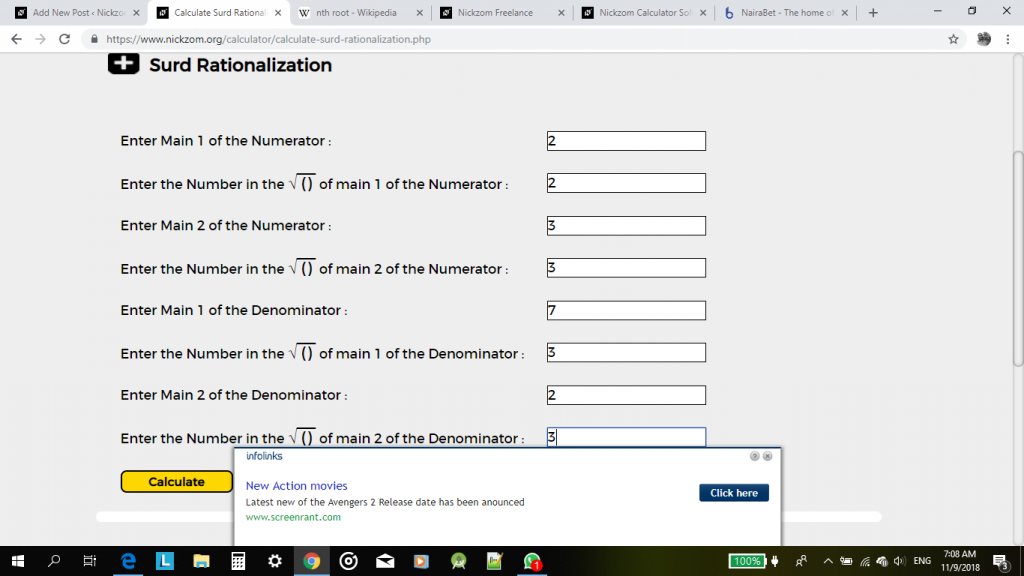Now, click on calculate to display the result.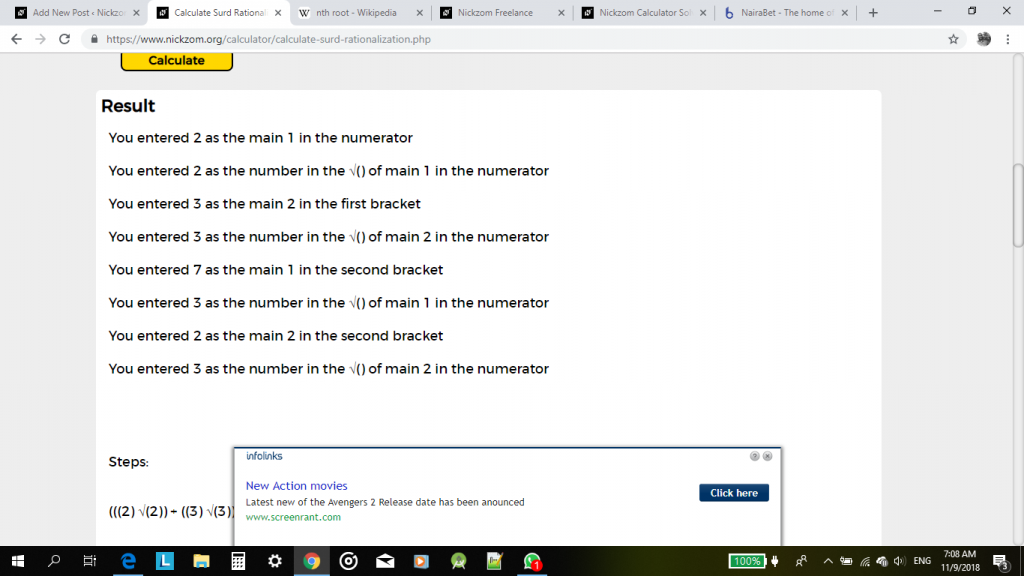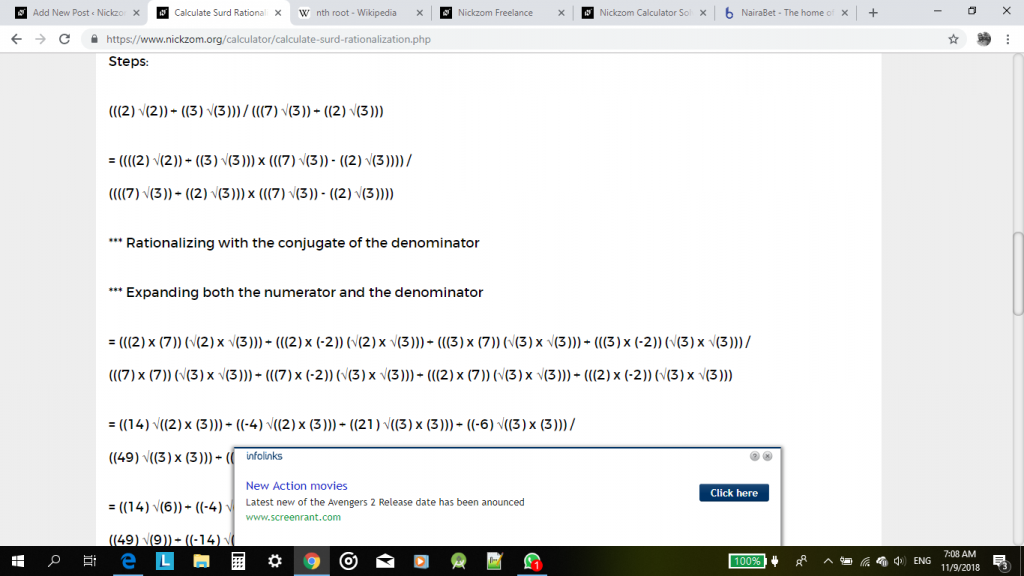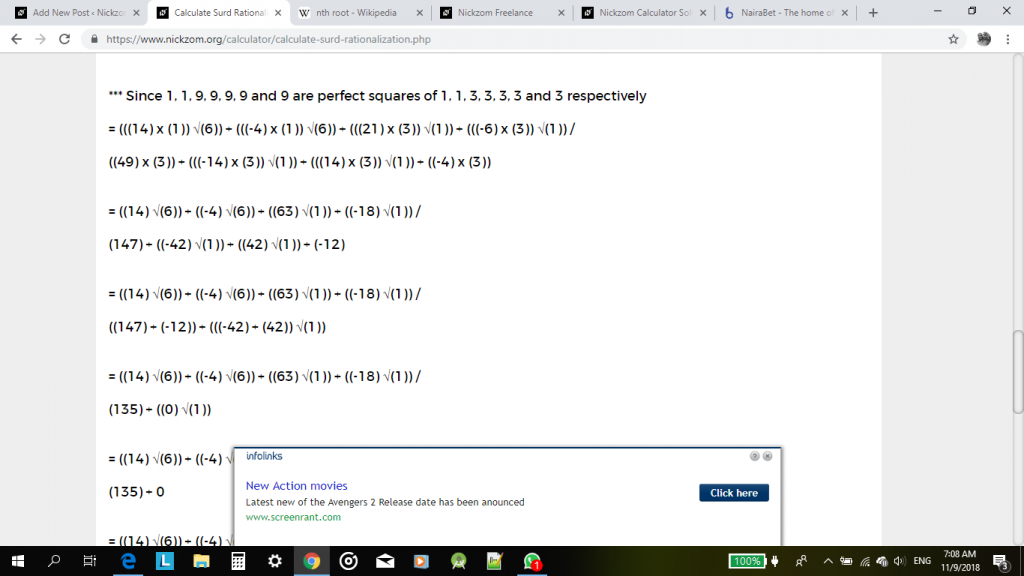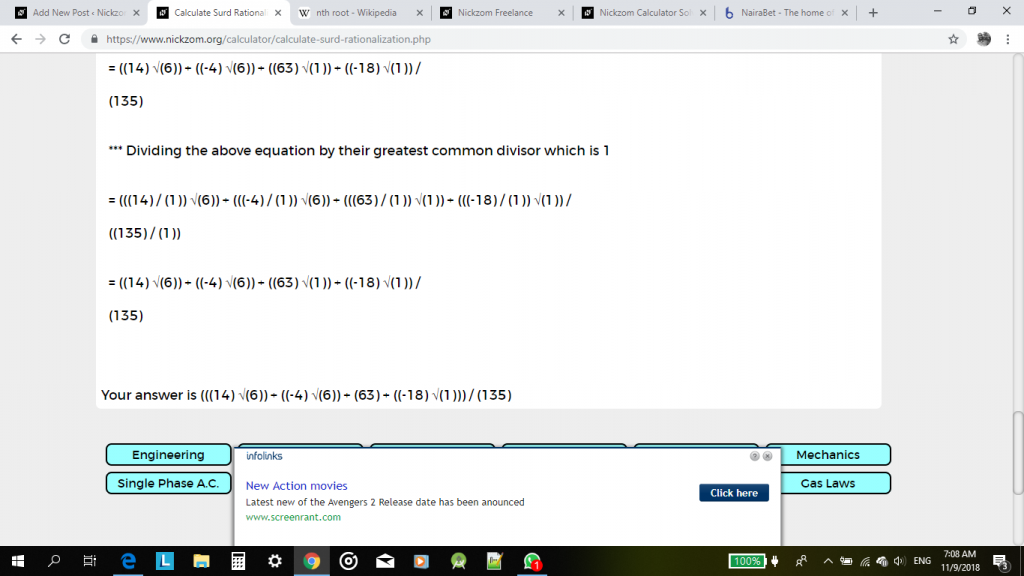I have seen that this steps and procedures might me cumbersome and a bit confusing for a reader or user. Therefore, an update would be coming soon with a new format of solving surd operations that can be easily understood and analysed. For now, with a bit of effort it could be understood or interpreted too.

Watch Out for the Update, Guys!

## Physics, Elasticity Calculations Solutions By Nickzom Calculator – The Calculator Encyclopedia

According to Wikipedia,

In Physics, elasticity is the ability of a body to resist a distorting influence and to return to its original size and shape when that influence or force is removed. Solid objects will deform when adequate forces are applied to them. If the material is elastic, the object will return to its initial shape and size when these forces are removed.

At Nickzom Calculator, we solve for the following:

1. Hooke’s Law Parameters (Force, Extension and Elastic Constant)
2. Young’s Modulus
3. Stress
4. Strain
5. Work Done or Elastic Potential Energy (Force)
6. Work Done or Elastic Potential Energy (Elastic Constant)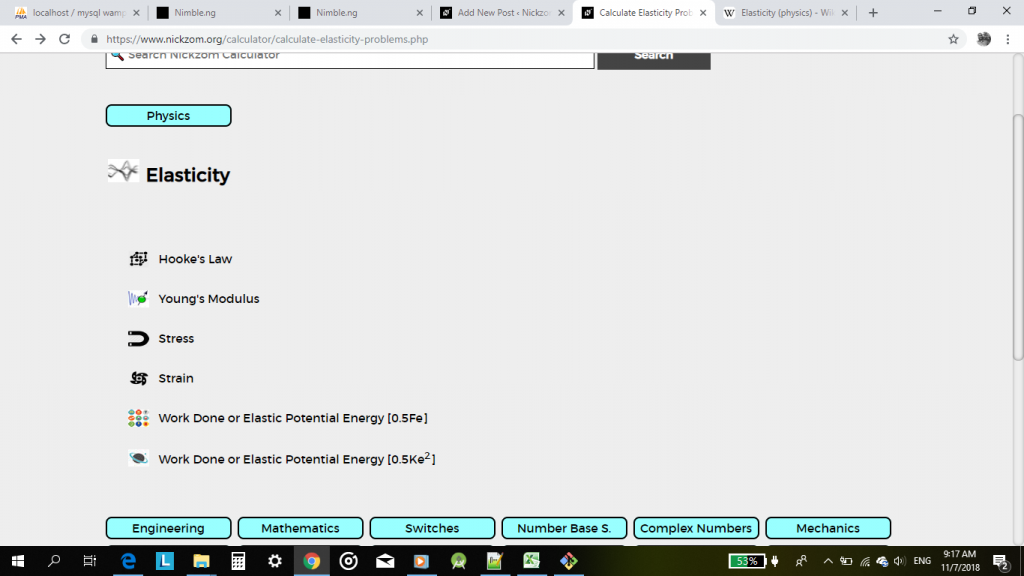Let’s say for example you want to find the force of an elastic material using Hooke’s law.

First, you need to get Nickzom Calculator either on web, or as an app via this links

Apple (iTunes – Paid) – https://itunes.apple.com/us/app/nickzom-calculator/id1331162702?mt=8

Next, you go to Physics, Then click on Elasticity.

Once you click on Elasticity, a new page comes up, click on Hooke’s lawEnter the elastic constant (K) and the extension for the elastic material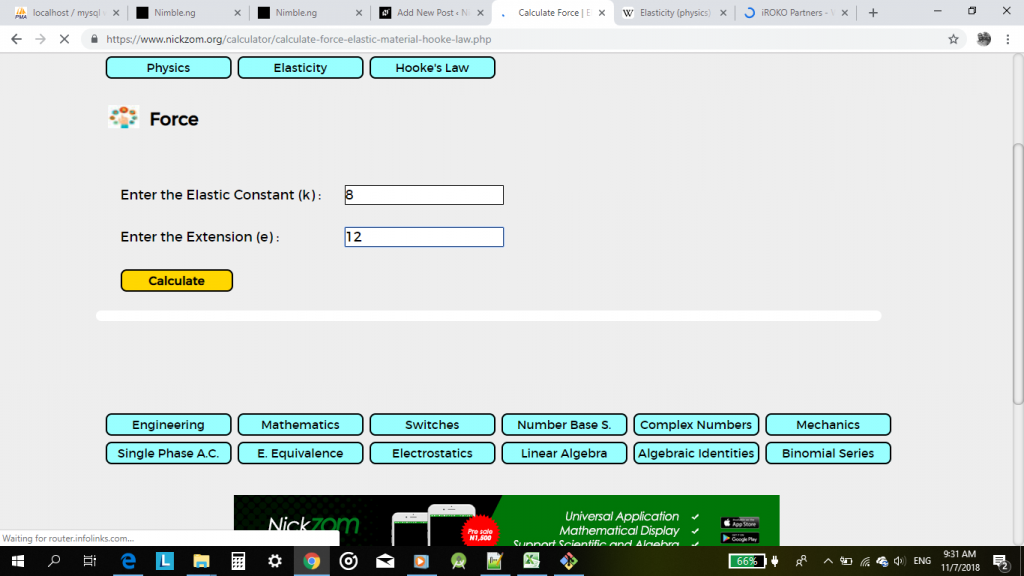Once, you have entered the values click on calculate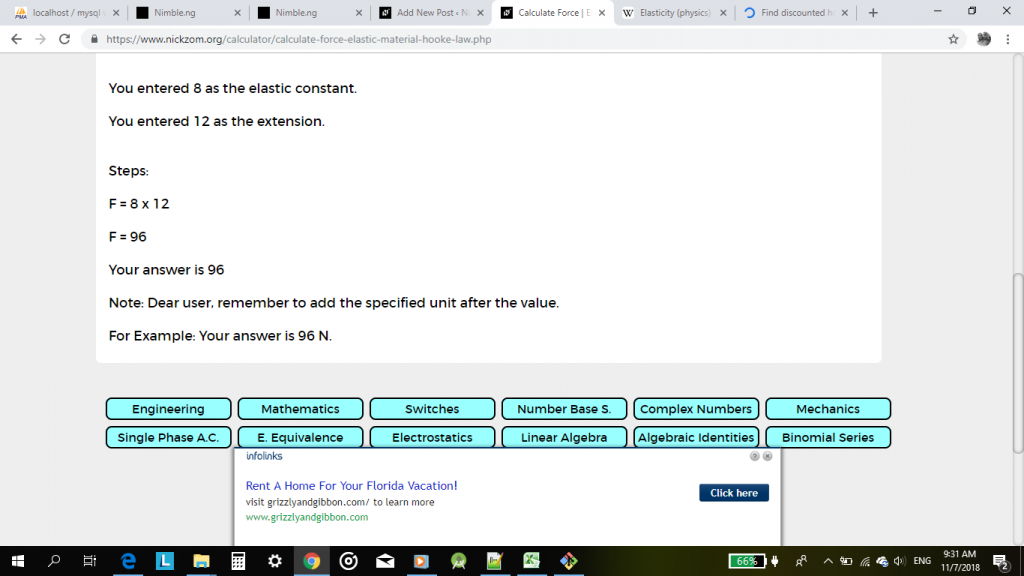As you can see above. Nickzom Calculator would solve the problems and provide you with a valid answer alongside the workings.

You can also apply the same steps in calculating for problems in Young’s modulus, stress, strain and work done.

## The Ovulation Calculator Working Now At Nickzom Calculator – The Calculator Encyclopedia

According to American Pregnancy,

Ovulation is when a mature egg is released from the ovary, pushed down the fallopian tube, and is made available to be fertilized.

Nickzom Calculator can solve and help you measure or rather inform you on your important cycle dates for on a subsequent of six cycles.

You can get this calculator on web for just NGN 1,500 per year or Google Play Store and Apple App Store for \$25.99.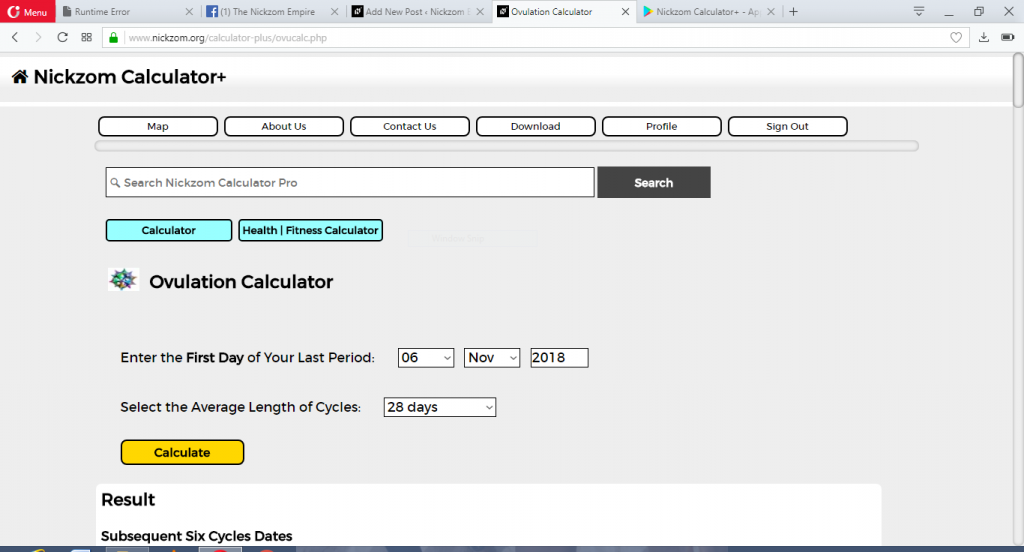Procedues:

First, open the app or website, select calculator and hit open section.

Scroll, to Health | Fitness Calculator, click on it.

Click on Ovulation Calculator

Enter the first day of your last period

Select your average length of cycles

Lastly, click on calculateOn pressing calculate, your approximate dates would be on display for your subsequent six cycles

The dates are:

• Most Probable Ovulation Date
• Ovulation Window
• Fertile Window
• Next Period
• Pregnancy Test
• Due Date If Pregnant

There are a few things you should know in this area

1. It is possible to experience bleeding without ovulating.
2. The odds on conceiving on the day of your ovulation is very tricky.
3. The best time to get pregnant is 2 days before ovulation
4. Egg cells survive for 12 to 24 hours in a woman’s body.